# Chapter 2 Data and Plots

## 2.2 The basic ingredients of R: variables and assignment

# examples of simple assignment
x <- 5
y <- 4
# the variables can be used in other operations
x+y
 9
# including defining new variables
z <- x + y
z
 9
# which can then be passed to other functions
sqrt(z)
 3
# example of Vector assignment
tree.heights <- c(4.3,7.1,6.3,5.2,3.2,2.1)
tree.heights
 4.3 7.1 6.3 5.2 3.2 2.1
tree.heights**2
 18.49 50.41 39.69 27.04 10.24  4.41
sum(tree.heights)
 28.2
mean(tree.heights)
 4.7
max.height <- max(tree.heights)
max.height
 7.1
tree.heights
 4.3 7.1 6.3 5.2 3.2 2.1
tree.heights     # first element
 4.3
tree.heights[1:3]   # a subset of elements 1 to 3
 4.3 7.1 6.3
sqrt(tree.heights[1:3]) #square roots of the subset
 2.073644 2.664583 2.509980
tree.heights[c(5,3,2)]  # a subset of elements 5,3,2: note the ordering
 3.2 6.3 7.1
# examples of Character Variable assignment
name <- "Lex Comber"
name
 "Lex Comber"
# these can be assigned to a vector of character variables
cities <- c("Leicester","Newcastle","London","Leeds","Exeter")
cities
 "Leicester" "Newcastle" "London"    "Leeds"
 "Exeter"   
length(cities)
 5
# an example of a Logical Variable
northern <- c(FALSE, TRUE, FALSE, TRUE, FALSE)
northern
 FALSE  TRUE FALSE  TRUE FALSE
# this can be used to subset other variables
cities[northern]
 "Newcastle" "Leeds"    

## 2.3 Data types and Data classes

### 2.3.1 Data Types in R

#### 2.3.1.1 Characters

character(8) 
 "" "" "" "" "" "" "" ""
# conversion
as.character("8") 
 "8"
# tests
is.character(8)
 FALSE
is.character("8")
 TRUE

#### 2.3.1.2 Numeric

numeric(8)
 0 0 0 0 0 0 0 0
# conversions
as.numeric(c("1980","-8","Geography"))
 1980   -8   NA
as.numeric(c(FALSE,TRUE))
 0 1
# tests
is.numeric(c(8, 8))
 TRUE
is.numeric(c(8, 8, 8, "8"))
 FALSE

#### 2.3.1.3 Logical

logical(7)
 FALSE FALSE FALSE FALSE FALSE FALSE FALSE
# conversion
as.logical(c(7, 5, 0, -4,5))
  TRUE  TRUE FALSE  TRUE  TRUE
# TRUE and FALSE can be converted to 1 and 0
as.logical(c(7,5,0,-4,5)) * 1
 1 1 0 1 1
as.logical(c(7,5,0,-4,5)) + 0
 1 1 0 1 1
# different ways to declare TRUE and FALSE
as.logical(c("True","T","FALSE","Raspberry","9","0", 0))
  TRUE  TRUE FALSE    NA    NA    NA    NA
data <- c(3, 6, 9, 99, 54, 32, -102)
# a logical test
index <- (data > 10)
index
 FALSE FALSE FALSE  TRUE  TRUE  TRUE FALSE
# used to subset data
data[index]
 99 54 32
sum(data)
 101
sum(data[index])
 185

### 2.3.2 Data Classes in R

#### 2.3.2.1 Vectors

# defining vectors
vector(mode = "numeric", length = 8)
 0 0 0 0 0 0 0 0
vector(length = 8)
 FALSE FALSE FALSE FALSE FALSE FALSE FALSE FALSE
# testing and conversion
tmp <- data.frame(a=10:15, b=15:20)
is.vector(tmp)
 FALSE
as.vector(tmp)
   a  b
1 10 15
2 11 16
3 12 17
4 13 18
5 14 19
6 15 20

#### 2.3.2.2 Matrices

# defining matrices
matrix(ncol = 2, nrow = 0)
     [,1] [,2]
matrix(1:6)
     [,1]
[1,]    1
[2,]    2
[3,]    3
[4,]    4
[5,]    5
[6,]    6
matrix(1:6, ncol = 2)
     [,1] [,2]
[1,]    1    4
[2,]    2    5
[3,]    3    6
# conversion and test
as.matrix(6:3)
     [,1]
[1,]    6
[2,]    5
[3,]    4
[4,]    3
is.matrix(as.matrix(6:3))
 TRUE
flow <- matrix(c(2000, 1243, 543, 1243, 212, 545,
654, 168, 109), c(3,3), byrow=TRUE)
# Rows and columns can have names, not just 1,2,3,...
colnames(flow) <- c("Leeds", "Maynooth"," Elsewhere")
rownames(flow) <- c("Leeds", "Maynooth", "Elsewhere")
# examine the matrix
flow 
          Leeds Maynooth  Elsewhere
Leeds      2000     1243        543
Maynooth   1243      212        545
Elsewhere   654      168        109
# and functions exist to summarise
outflows <- rowSums(flow)
outflows
    Leeds  Maynooth Elsewhere
3786      2000       931 
z <- c(6,7,8)
names(z) <- c("Newcastle","London","Manchester")
z
 Newcastle     London Manchester
6          7          8 
?sum
help(sum)
# Create a variable to pass to other summary functions
x <- matrix(c(3,6,8,8,6,1,-1,6,7),c(3,3),byrow=TRUE)
# Sum over rows
rowSums(x)
# Sum over columns
colSums(x)
# Calculate column means
colMeans(x)
# Apply function over rows (1) or columns (2) of x
apply(x,1,max)
# Logical operations to select matrix elements
x[,c(TRUE,FALSE,TRUE)]
# Add up all of the elements in x
sum(x)
# Pick out the leading diagonal
diag(x)
# Matrix inverse
solve(x)
# Tool to handle rounding
zapsmall(x %*% solve(x))   

#### 2.3.2.3 Factors

# a vector assignment
house.type <- c("Bungalow", "Flat", "Flat",
"Detached", "Flat", "Terrace", "Terrace")
# a factor assignment
house.type <- factor(c("Bungalow", "Flat",
"Flat", "Detached", "Flat", "Terrace", "Terrace"),
levels=c("Bungalow","Flat","Detached","Semi","Terrace"))
house.type
 Bungalow Flat     Flat     Detached Flat     Terrace
 Terrace
Levels: Bungalow Flat Detached Semi Terrace
# table can be used to summarise
table(house.type)
house.type
Bungalow     Flat Detached     Semi  Terrace
1        3        1        0        2 
# 'levels' control what can be assigned
house.type <- factor(c("People Carrier", "Flat",
"Flat", "Hatchback", "Flat", "Terrace", "Terrace"),
levels=c("Bungalow","Flat","Detached","Semi","Terrace"))
house.type
 <NA>    Flat    Flat    <NA>    Flat    Terrace Terrace
Levels: Bungalow Flat Detached Semi Terrace

#### 2.3.2.4 Ordering

income <-factor(c("High", "High", "Low", "Low",
"Low", "Medium", "Low", "Medium"),
levels=c("Low", "Medium", "High"))
income > "Low"
 NA NA NA NA NA NA NA NA
# 'levels' in 'ordered' defines a relative order
income <-ordered(c("High", "High", "Low", "Low",
"Low", "Medium", "Low", "Medium"),
levels=c("Low", "Medium", "High"))
income > "Low"
  TRUE  TRUE FALSE FALSE FALSE  TRUE FALSE  TRUE
sort(income)

#### 2.3.2.5 Lists

tmp.list <- list("Lex Comber",c(2015, 2018),
"Lecturer", matrix(c(6,3,1,2), c(2,2)))
tmp.list
[]
 "Lex Comber"

[]
 2015 2018

[]
 "Lecturer"

[]
[,1] [,2]
[1,]    6    1
[2,]    3    2
# elements of the list can be selected
tmp.list[]
     [,1] [,2]
[1,]    6    1
[2,]    3    2
employee <- list(name="Lex Comber", start.year = 2015,
position="Professor")
employee
$name  "Lex Comber"$start.year
 2015

$position  "Professor" append(tmp.list, list(c(7,6,9,1))) # lappy with different functions lapply(tmp.list[], is.numeric) lapply(tmp.list, length) #### 2.3.2.6 Defining your own Classes employee <- list(name="Lex Comber", start.year = 2015, position="Professor") class(employee) <- "staff" print.staff <- function(x) { cat("Name: ",x$name,"\n")
cat("Start Year: ",x$start.year,"\n") cat("Job Title: ",x$position,"\n")}
# an example of the print class
print(employee)
Name:  Lex Comber
Start Year:  2015
Job Title:  Professor 
print(unclass(employee))
$name  "Lex Comber"$start.year
 2015

$position  "Professor" #### 2.3.2.7 Classes in Lists new.staff <- function(name,year,post) { result <- list(name=name, start.year=year, position=post) class(result) <- "staff" return(result)} leeds.uni <- vector(mode='list',3) # assign values to elements in the list leeds.uni[] <- new.staff("Heppenstall, Alison", 2017,"Professor") leeds.uni[] <- new.staff("Comber, Lex", 2015,"Professor") leeds.uni[] <- new.staff("Langlands, Alan", 2014,"VC") And the list can be examined by entering: leeds.uni #### 2.3.2.8data.frame vs tibble df <- data.frame(dist = seq(0,400, 100), city = c("Leeds", "Nottingham", "Leicester", "Durham", "Newcastle")) str(df) 'data.frame': 5 obs. of 2 variables:$ dist: num  0 100 200 300 400
$city: Factor w/ 5 levels "Durham","Leeds",..: 2 5 3 1 4 df$city
df <- data.frame(dist = seq(0,400, 100),
city = c("Leeds", "Nottingham", "Leicester", "Durham", "Newcastle"),
stringsAsFactors = FALSE)
str(df)
'data.frame':   5 obs. of  2 variables:
$dist: num 0 100 200 300 400$ city: chr  "Leeds" "Nottingham" "Leicester" "Durham" ...
tb <- tibble(dist = seq(0,400, 100),
city = c("Leeds", "Nottingham", "Leicester", "Durham", "Newcastle"))
df$ci  "Leeds" "Nottingham" "Leicester" "Durham"  "Newcastle"  tb$ci
NULL
# 1 column
df[,2]
tb[,2]
class(df[,2])
class(tb[,2])
# 2 columns
df[,1:2]
tb[,1:2]
class(df[,1:2])
class(tb[,1:2])
data.frame(tb)
as_tibble(df) 
cbind(df, Pop = c(700,250,230,150,1200))
  dist       city  Pop
1    0      Leeds  700
2  100 Nottingham  250
3  200  Leicester  230
4  300     Durham  150
5  400  Newcastle 1200
cbind(tb, Pop = c(700,250,230,150,1200))
  dist       city  Pop
1    0      Leeds  700
2  100 Nottingham  250
3  200  Leicester  230
4  300     Durham  150
5  400  Newcastle 1200
vignette("tibble")

### 2.3.3 Self-Test Questions

#### 2.3.3.1 Factors

colours <- factor(c("red","blue","red","white",
"silver","red","white","silver",
"red","red","white","silver","silver"),
levels=c("red","blue","white","silver","black"))

Self-Test Question 1:

colours <- "orange"
colours
colours <- factor(c("red","blue","red","white",
"silver","red","white","silver",
"red","red","white","silver","silver"),
levels=c("red","blue","white","silver","black"))
table(colours)
colours
red   blue  white silver  black
5      1      3      4      0 
colours2 <-c("red","blue","red","white",
"silver","red","white","silver",
"red","red","white","silver")
# Now, make the table
table(colours2)
colours2
blue    red silver  white
1      5      3      3 

Self-Test Question 2

car.type <- factor(c("saloon","saloon","hatchback",
"saloon","convertible","hatchback","convertible",
"saloon", "hatchback","saloon", "saloon",
"saloon","hatchback"),
levels=c("saloon","hatchback","convertible"))
table(car.type, colours)
             colours
car.type      red blue white silver black
saloon        2    1     2      2     0
hatchback     3    0     0      1     0
convertible   0    0     1      1     0
crosstab <- table(car.type,colours)

Self-Test Question 3

engine <- ordered(c("1.1litre","1.3litre","1.1litre",
"1.3litre","1.6litre","1.3litre","1.6litre",
"1.1litre","1.3litre","1.1litre", "1.1litre",
"1.3litre","1.3litre"),
levels=c("1.1litre","1.3litre","1.6litre"))
engine > "1.1litre"
  FALSE  TRUE FALSE  TRUE  TRUE  TRUE  TRUE FALSE  TRUE
 FALSE FALSE  TRUE  TRUE

Self-Test Question 4

#### 2.3.3.2 Matrices

dim(crosstab) # Matrix dimensions
 3 5
rowSums(crosstab) # Row sums
     saloon   hatchback convertible
7           4           2 
colnames(crosstab) # Column names
 "red"    "blue"   "white"  "silver" "black" 
apply(crosstab,1,max)
     saloon   hatchback convertible
2           3           1 
apply(crosstab,2,max)
   red   blue  white silver  black
3      1      2      2      0 
example <- c(1.4,2.6,1.1,1.5,1.2)
which.max(example)
 2

Self-Test Question 5

Self-Test Question 6

levels(engine)
 "1.1litre" "1.3litre" "1.6litre"
levels(colours)[which.max(crosstab[,1])]
 "blue"
colnames(crosstab)[which.max(crosstab[,1])]
 "blue"
colnames(crosstab)
 "red"    "blue"   "white"  "silver" "black" 
crosstab[,1]
     saloon   hatchback convertible
2           3           0 
which.max(crosstab[,1])
hatchback
2 
# Defines the function
which.max.name <- function(x) {
return(names(x)[which.max(x)])}
# Next, give the variable 'example' names for the values
"Harrogate","Thirsk")
example
 Bradford     Leeds      York Harrogate    Thirsk
1.4       2.6       1.1       1.5       1.2 
which.max.name(example) 
 "Leeds"

Self Test Question 7

#### 2.3.3.3 Lists

var <- list(name1=value1, name2=value2, ...)

Self Test Question 8

#### 2.3.3.4 Classes

new.sales.data <- function(colours, car.type) {
xtab <- table(car.type,colours)
result <- list(colour=apply(xtab,1,which.max.name),
type=apply(xtab,2,which.max.name),
total=sum(xtab))
class(result) <- "sales.data"
return(result)}
this.week <- new.sales.data(colours,car.type)
this.week
$colour saloon hatchback convertible "red" "red" "white"$type
red        blue       white      silver       black
"hatchback"    "saloon"    "saloon"    "saloon"    "saloon"

$total  13 attr(,"class")  "sales.data" Self Test question 9 ## 2.4 Plots ### 2.4.1 Basic Plot Tools x1 <- rnorm(100) y1 <- rnorm(100) plot(x1,y1)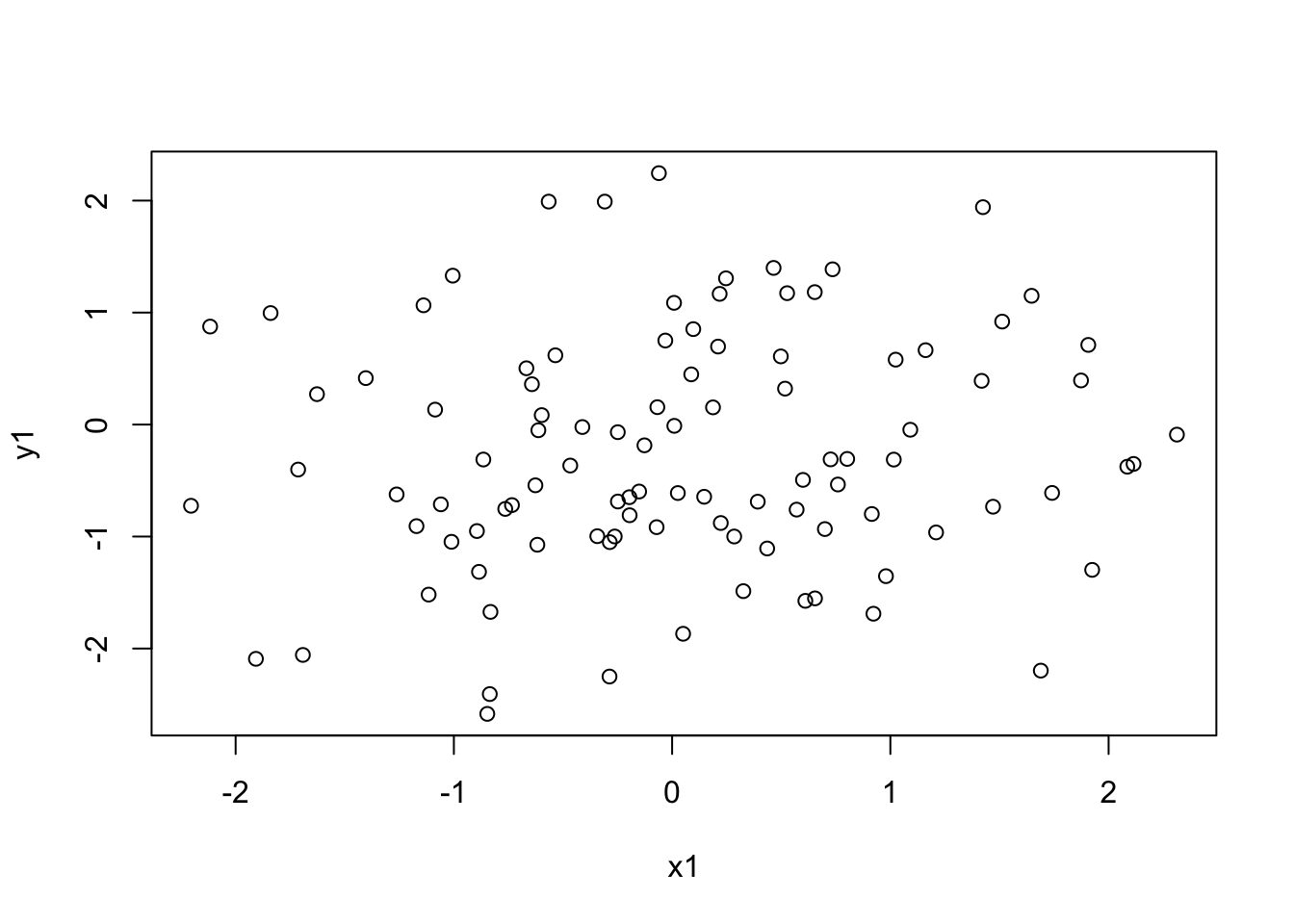Figure 2.1: A basic scatterplot plot(x1,y1,pch=16, col='red') x2 <- seq(0,2*pi,len=100) y2 <- sin(x2) plot(x2,y2,type='l') plot(x2,y2,type='l', lwd=3, col='darkgreen')  plot(x2,y2,type='l', col='darkgreen', lwd=3, ylim=c(-1.2,1.2)) y2r <- y2 + rnorm(100,0,0.1) points(x2,y2r, pch=16, col='darkred')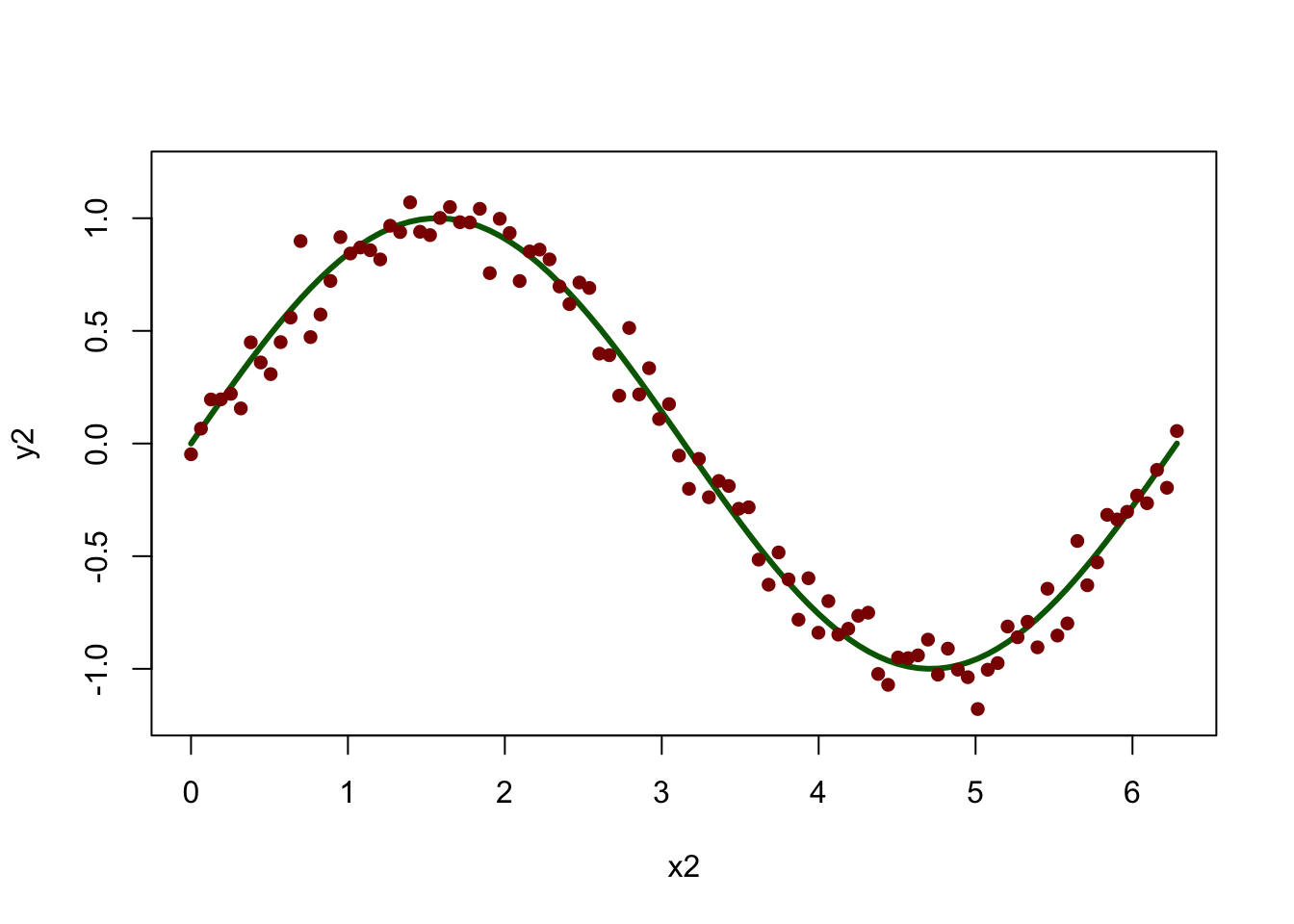Figure 2.2: A line plot with points added y4 <- cos(x2) plot(x2, y2, type='l', lwd=3, col='darkgreen') lines(x2, y4, lwd=3, lty=2, col='darkblue') x2 <- seq(0,2*pi,len=100) y2 <- sin(x2) y4 <- cos(x2) # specify the plot layout and order par(mfrow = c(1,2)) # plot #1 plot(y2,y4) polygon(y2,y4,col='lightgreen') # plot #2: this time with 'asp' to set the aspect ratio of the axes plot(y2,y4, asp=1, type='n') polygon(y2,y4,col='lightgreen')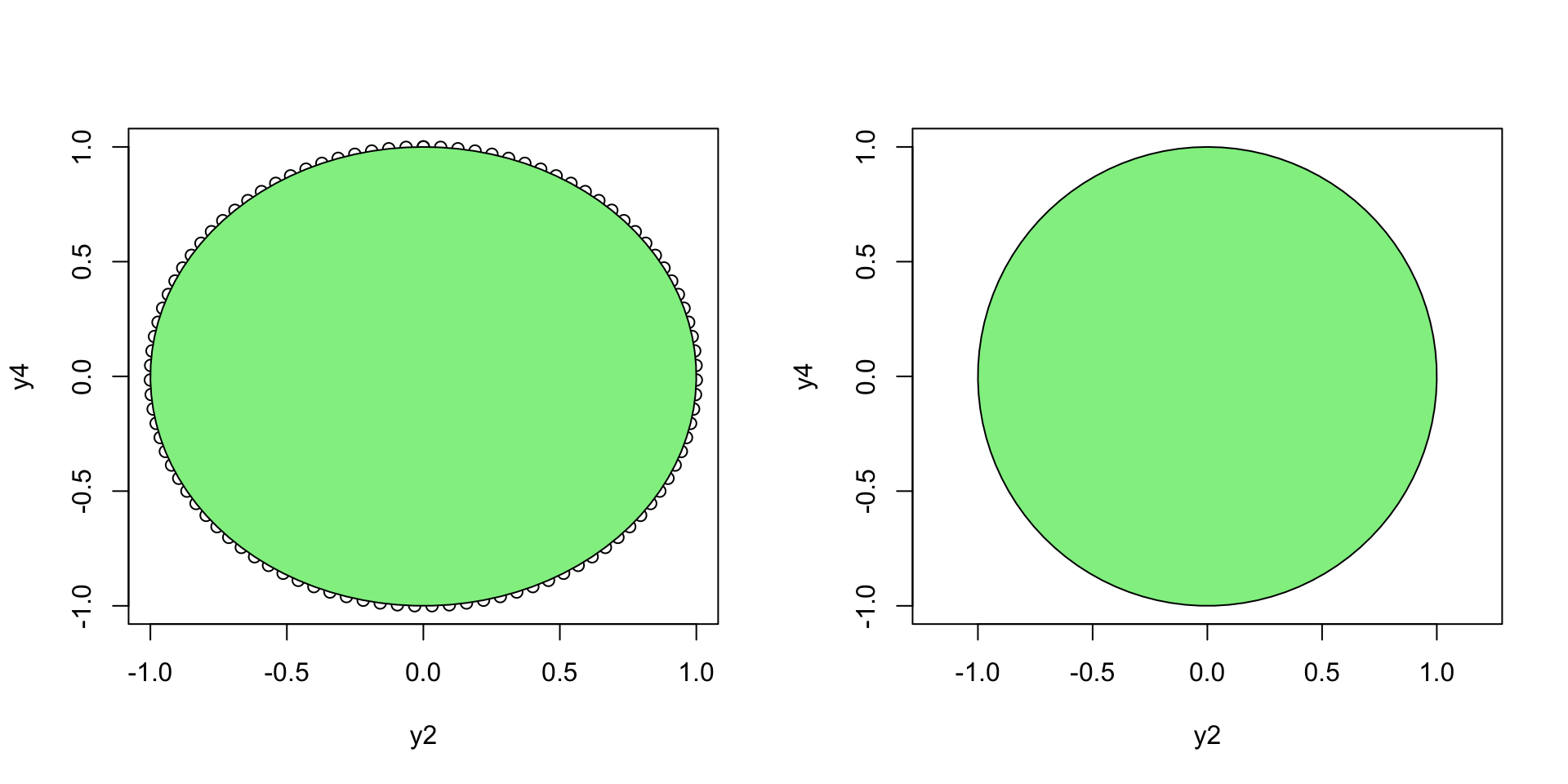Figure 2.3: Points with polygons added install.packages("GISTools", depend = T) library(GISTools) # library(GISTools) data(georgia) # select the first element appling <- georgia.polys[] # set the plot extent plot(appling, asp=1, type='n', xlab="Easting", ylab="Northing") # plot the selected features with hatching polygon(appling, density=14, angle=135)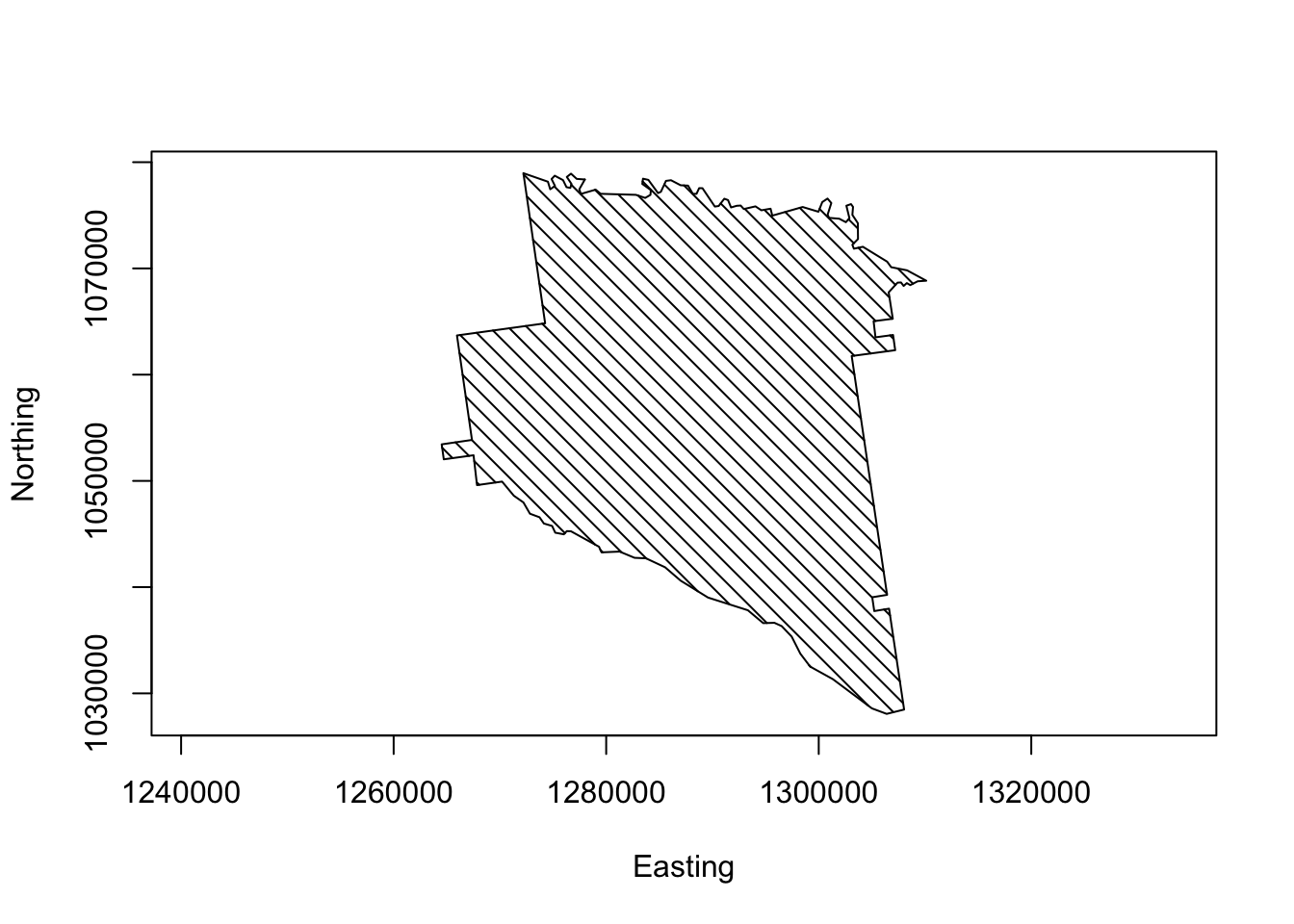Figure 2.4: Appling County plotted from coordinate pairs ### 2.4.2 Plot colours colours() plot(appling, asp=1, type='n', xlab="Easting", ylab="Northing") polygon(appling, col=rgb(0,0.5,0.7)) polygon(appling, col=rgb(0,0.5,0.7,0.4)) # set the plot extent plot(appling, asp=1, type='n', xlab="Easting", ylab="Northing") # plot the points points(x = runif(500,126,132)*10000, y = runif(500,103,108)*10000, pch=16, col='red') # plot the polygon with a transparency factor polygon(appling, col=rgb(0,0.5,0.7,0.4))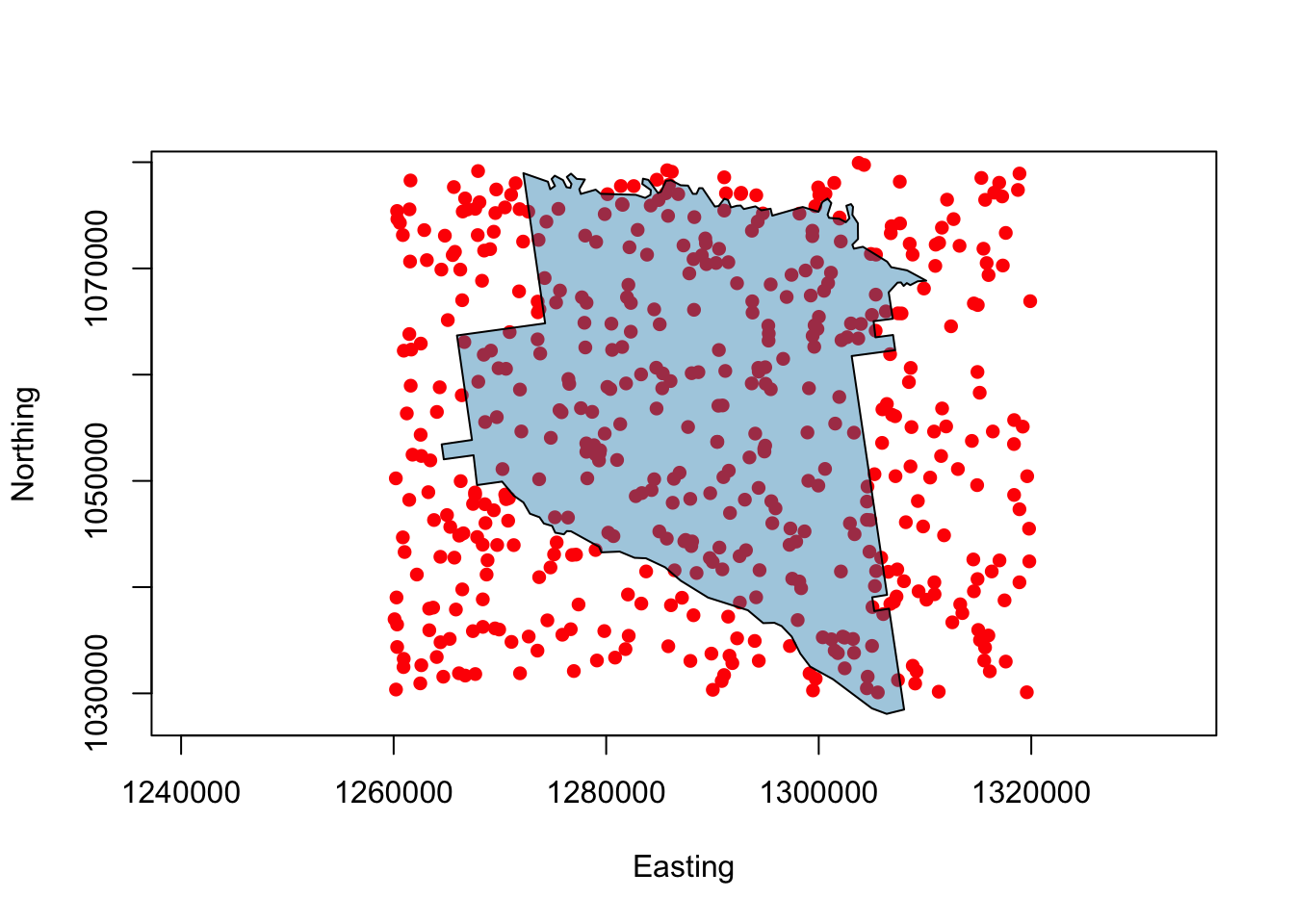Figure 2.5: Appling County with transparency plot(appling, asp=1, type='n', xlab="Easting", ylab="Northing") polygon(appling, col="#B3B333") # add text, sepcifying its placement, colour and size text(1287000,1053000,"Appling County",cex=1.5) text(1287000,1049000,"Georgia",col='darkred')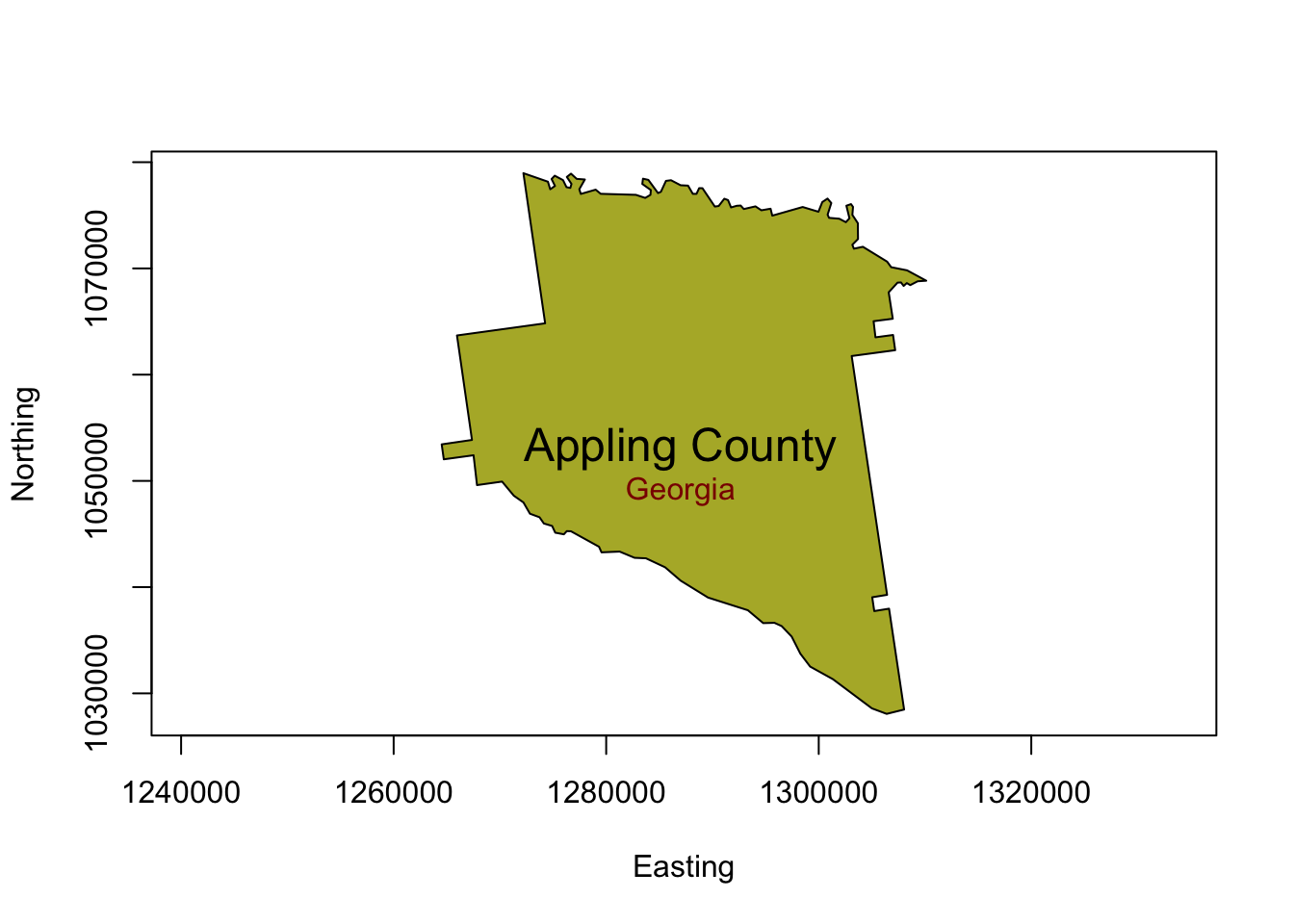Figure 2.6: Appling County with text plot(c(-1.5,1.5),c(-1.5,1.5),asp=1, type='n') # plot the green/blue rectangle rect(-0.5,-0.5,0.5,0.5, border=NA, col=rgb(0,0.5,0.5,0.7)) # then the second one rect(0,0,1,1, col=rgb(1,0.5,0.5,0.7))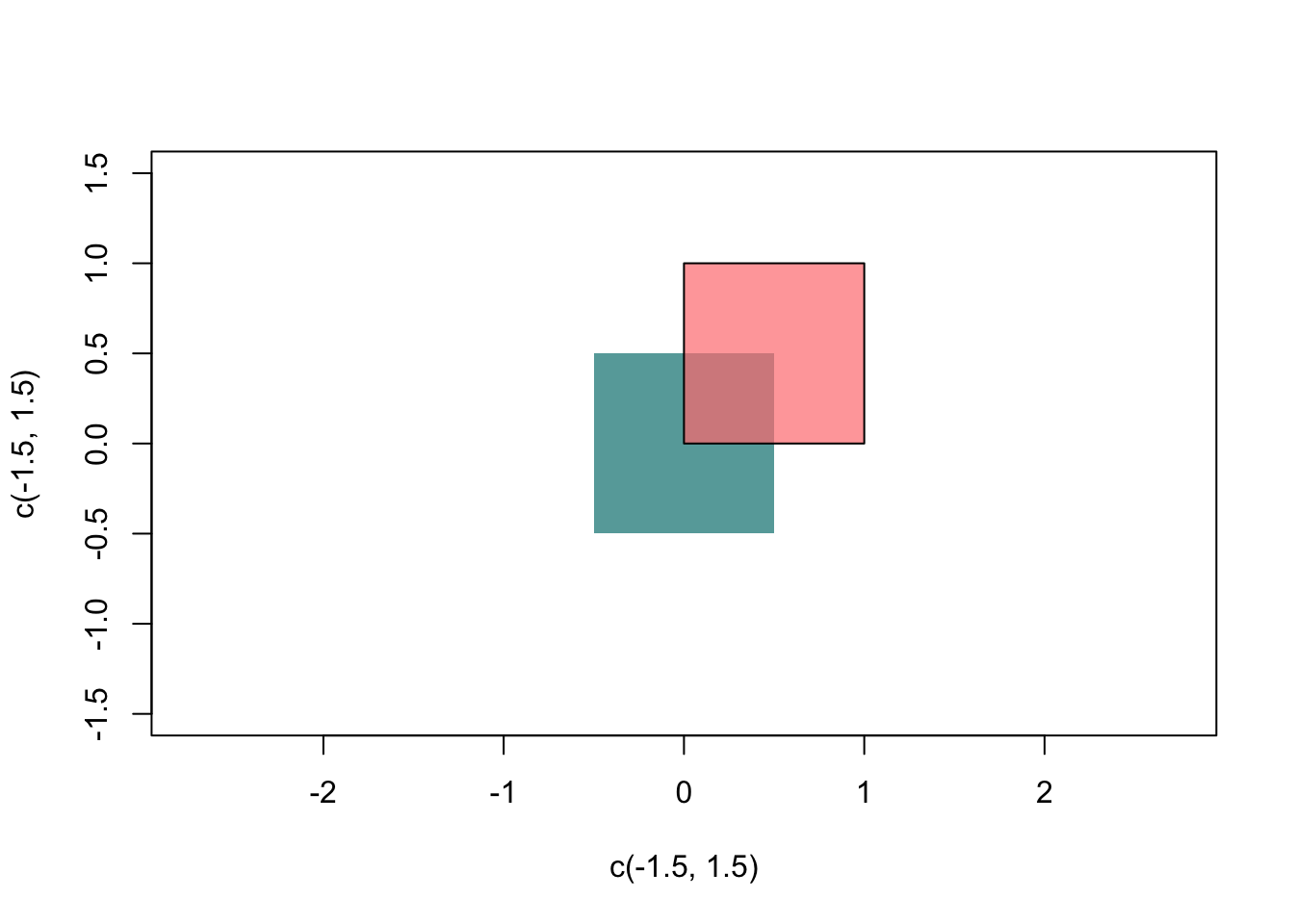Figure 2.7: Plotting rectangles # load some grid data data(meuse.grid) # define a SpatialPixelsDataFrame from the data mat = SpatialPixelsDataFrame(points = meuse.grid[c("x", "y")], data = meuse.grid) # set some plot parameters (1 row, 2 columns) par(mfrow = c(1,2)) # set the plot margins par(mar = c(0,0,0,0)) # plot the points using the default shading image(mat, "dist") # load the package library(RColorBrewer) # select and examine a colour palette with 7 classes greenpal <- brewer.pal(7,'Greens') # and now use this to plot the data image(mat, "dist", col=greenpal)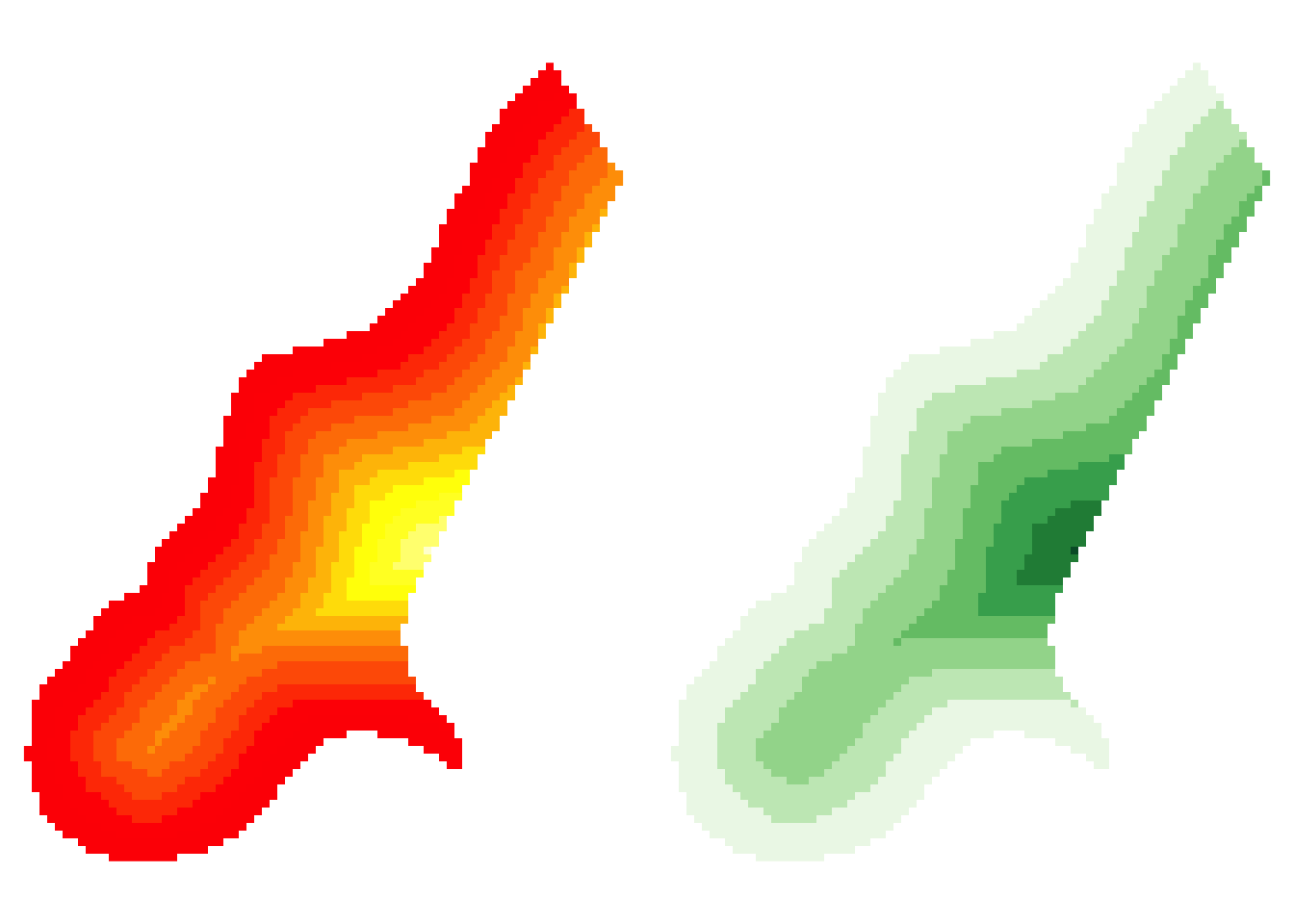Figure 2.8: Plotting raster data # reset par par(mfrow = c(1,1)) ## 2.5 Another plot option: ggplot ### 2.5.1 Introduction to ggplot install.packages("tidyverse", dep = T) install.packages("ggplot2", dep = T) library(ggplot2) qplot(x2,y2r,col=I('darkred'), ylim=c(-1.2, 1.2)) + geom_line(aes(x2,y2), col=I("darkgreen"), size = I(1.5)) + theme(axis.text=element_text(size=20), axis.title=element_text(size=20,face="bold"))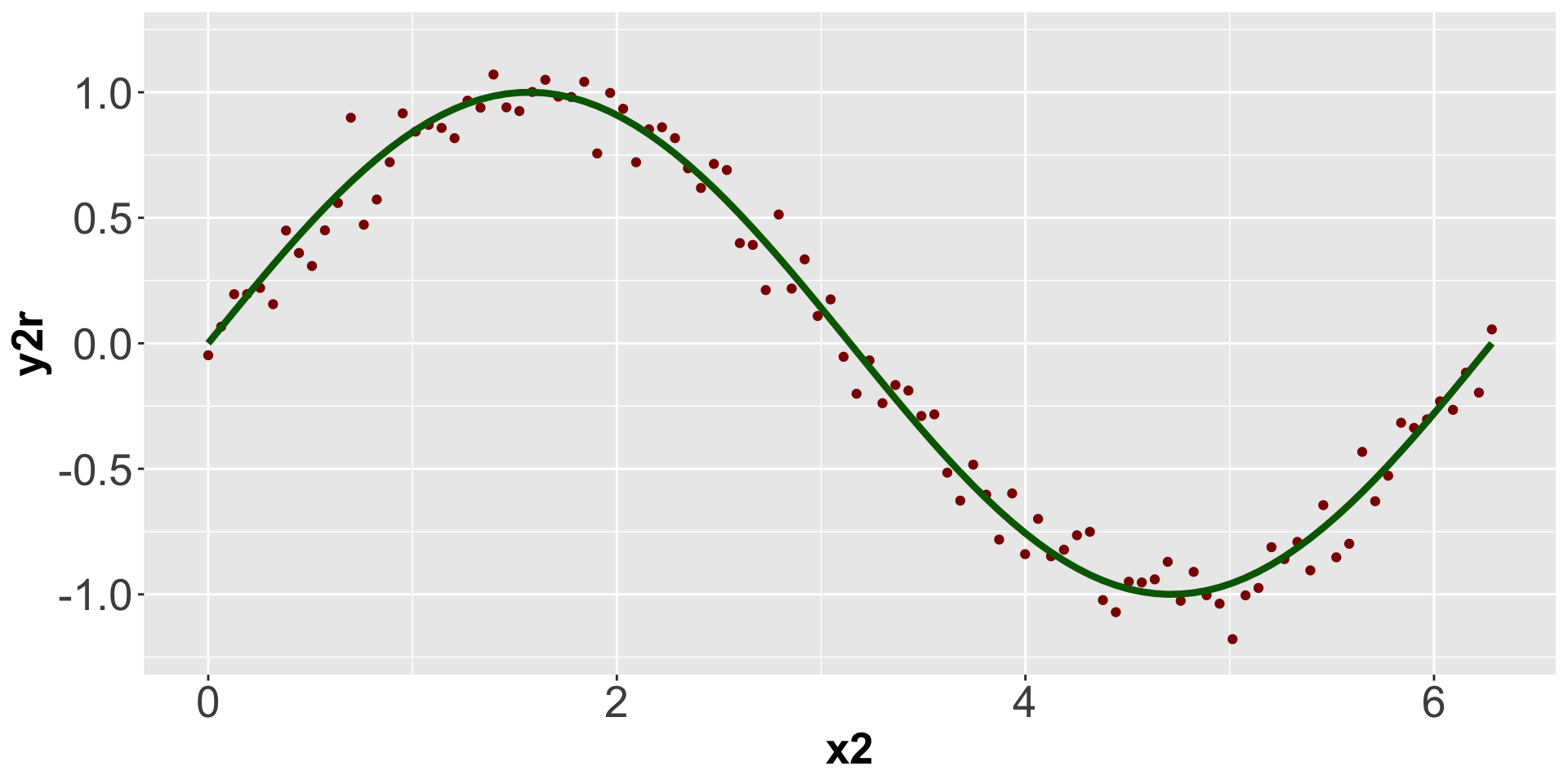Figure 2.9: A simple qplot  theme_bw()  theme_dark() appling <- data.frame(appling) colnames(appling) <- c("X", "Y") install.packages("gridExtra", dep = T) # create the first plot with qplot p1 <- qplot(X, Y, data = appling, geom = "polygon", asp = 1, colour = I("black"), fill=I(rgb(0,0.5,0.7,0.4))) + theme(axis.text=element_text(size=12), axis.title=element_text(size=20)) # create a data.frame to hold the points df <- data.frame(x = runif(500,126,132)*10000, y = runif(500,103,108)*10000) # now use ggplot to contruct the layers of the plot p2 <- ggplot(appling, aes(x = X, y= Y)) + geom_polygon(fill = I(rgb(0,0.5,0.7,0.4))) + geom_point(data = df, aes(x, y),col=I('red')) + coord_fixed() + theme(axis.text=element_text(size=12), axis.title=element_text(size=20)) # finally combine these in a single plot # using the grid.arrange function # NB you may have to install the gridExtra package library(gridExtra) grid.arrange(p1, p2, ncol = 2)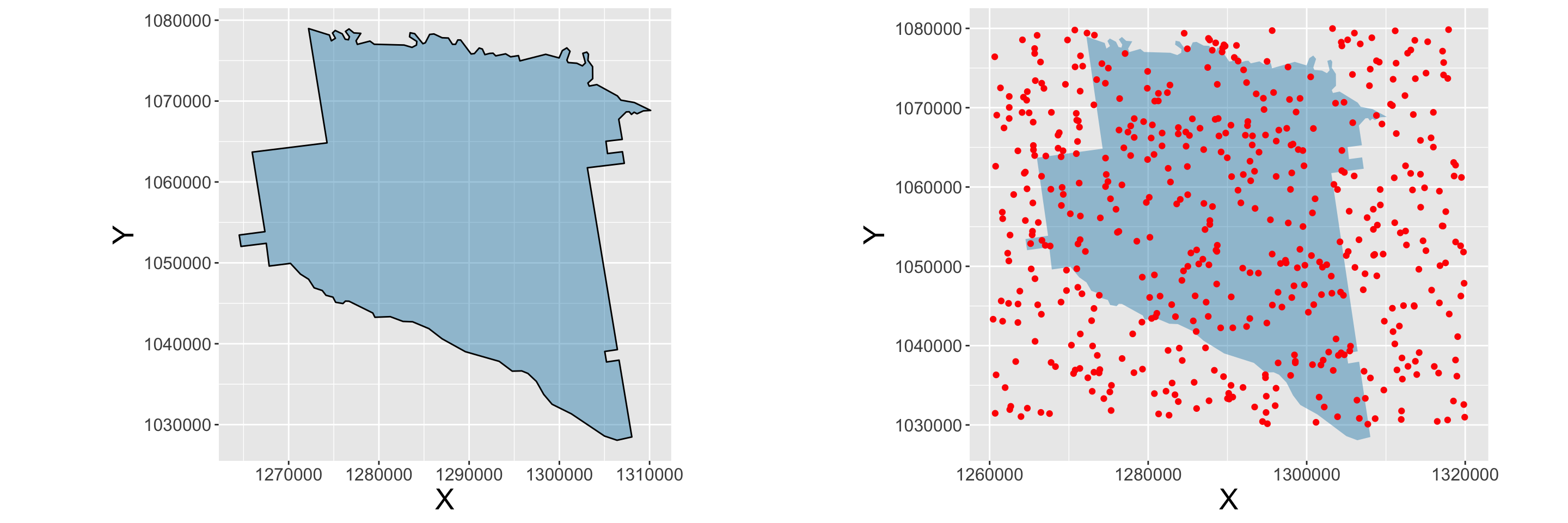Figure 2.10: A simple qplot of a polygon ### 2.5.2 Different ggplot types # data.frame df <- data.frame(georgia) # tibble tb <- as.tibble(df) tb tb$rural <- as.factor((tb$PctRural > 50) + 0) levels(tb$rural) <- list("Non-Rural" = 0, "Rural"=1)
tb$IncClass <- rep("Average", nrow(tb)) tb$IncClass[tb$MedInc >= 41204] = "Rich" tb$IncClass[tb$MedInc <= 29773] = "Poor" table(tb$IncClass)
ggplot(data = tb, mapping=aes(x=PctBach, y=PctEld)) +
geom_point()
ggplot(data = tb, mapping=aes(x=PctBach, y=PctEld, colour=rural)) +
geom_point()
ggplot(data = tb, mapping = aes(x = PctBach, y = PctEld)) +
geom_point() +
geom_smooth(method = "lm")
ggplot(data = tb, mapping = aes(x = PctBach, y = PctEld)) +
geom_point() +
geom_smooth(method = "lm", col = "red", fill = "lightsalmon") +
theme_bw() +
xlab("% of population with bachelor degree") +
ylab("% of population that are elderly")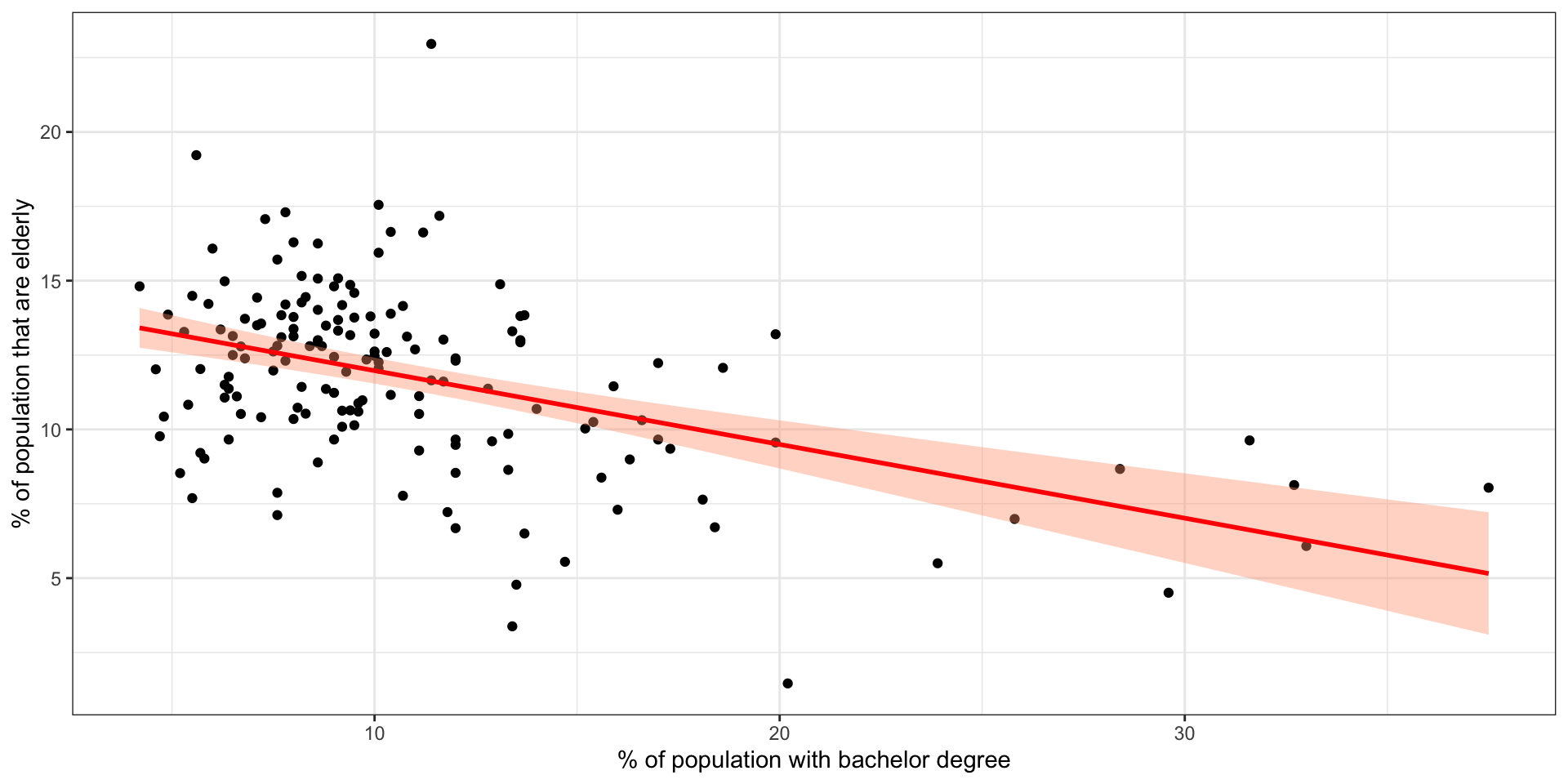Figure 2.11: A ggplot scatterplot

ggplot(tb, aes(x=MedInc)) +
geom_histogram(, binwidth = 5000, colour = "red", fill = "grey")
ggplot(tb, aes(x=MedInc)) +
geom_histogram(aes(y=..density..),
binwidth=5000,colour="white") +
geom_density(alpha=.4, fill="darksalmon") +
# Ignore NA values for mean
geom_vline(aes(xintercept=median(MedInc, na.rm=T)),
color="orangered1", linetype="dashed", size=1)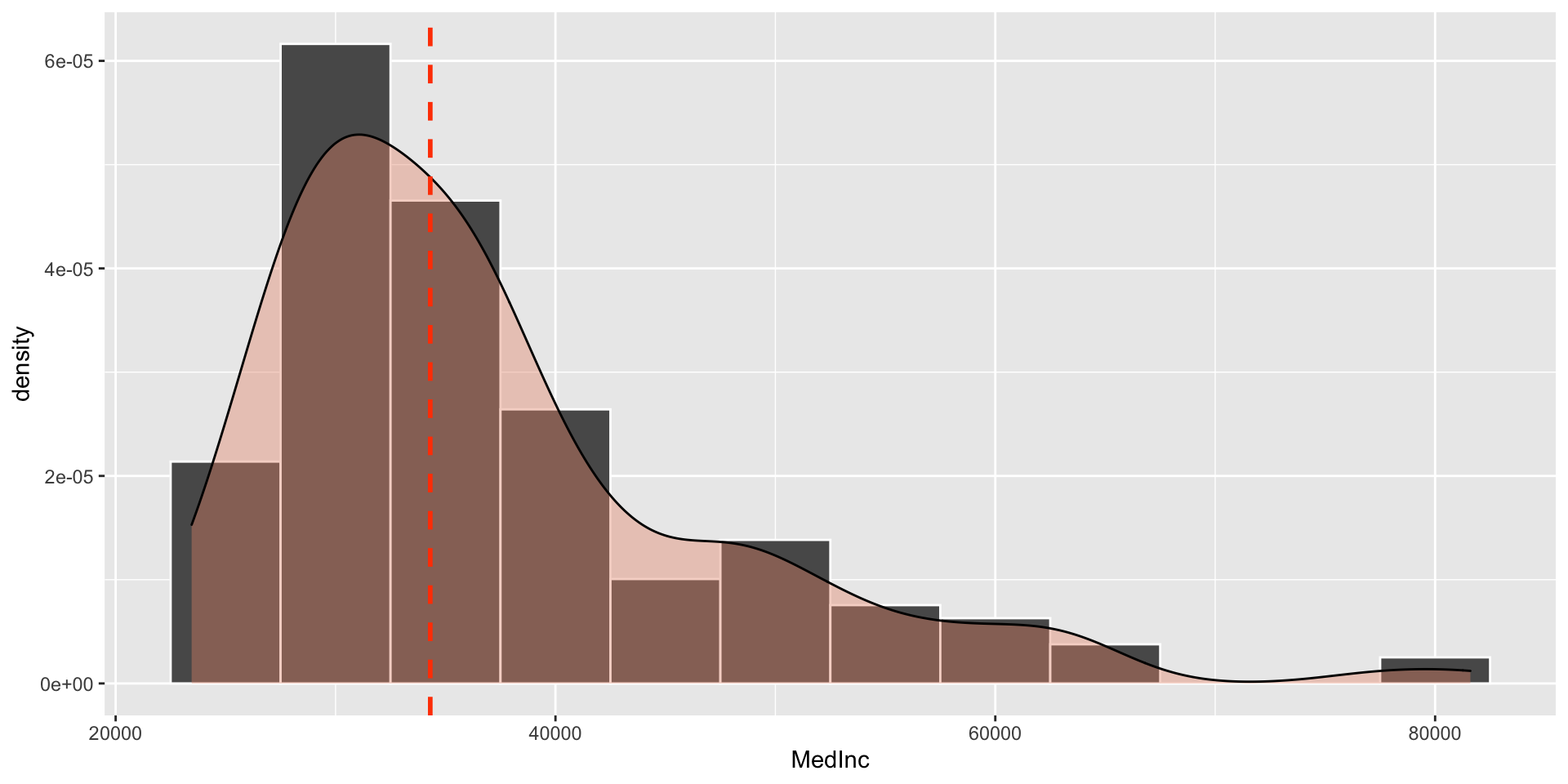Figure 2.12: A ggplot density histogram

ggplot(tb, aes(x=PctBach, fill=IncClass)) +
geom_histogram(color="grey30",
binwidth = 1) +
scale_fill_manual("Income Class",
values = c("orange", "palegoldenrod","firebrick3")) +
facet_grid(IncClass~.) +
xlab("% Bachelor degrees") +
ggtitle("Bachelors degree % in different income classes")
gplot(tb, aes(x = "",PctBach)) +
geom_boxplot() 
ggplot(tb, aes(IncClass, PctBach, fill = factor(rural))) +
geom_boxplot() +
scale_fill_manual(name = "Rural",
values = c("orange", "firebrick3"),
labels = c("Non-Rural"="Not Rural","Rural"="Rural")) +
xlab("Income Class") +
ylab("% Bachelors")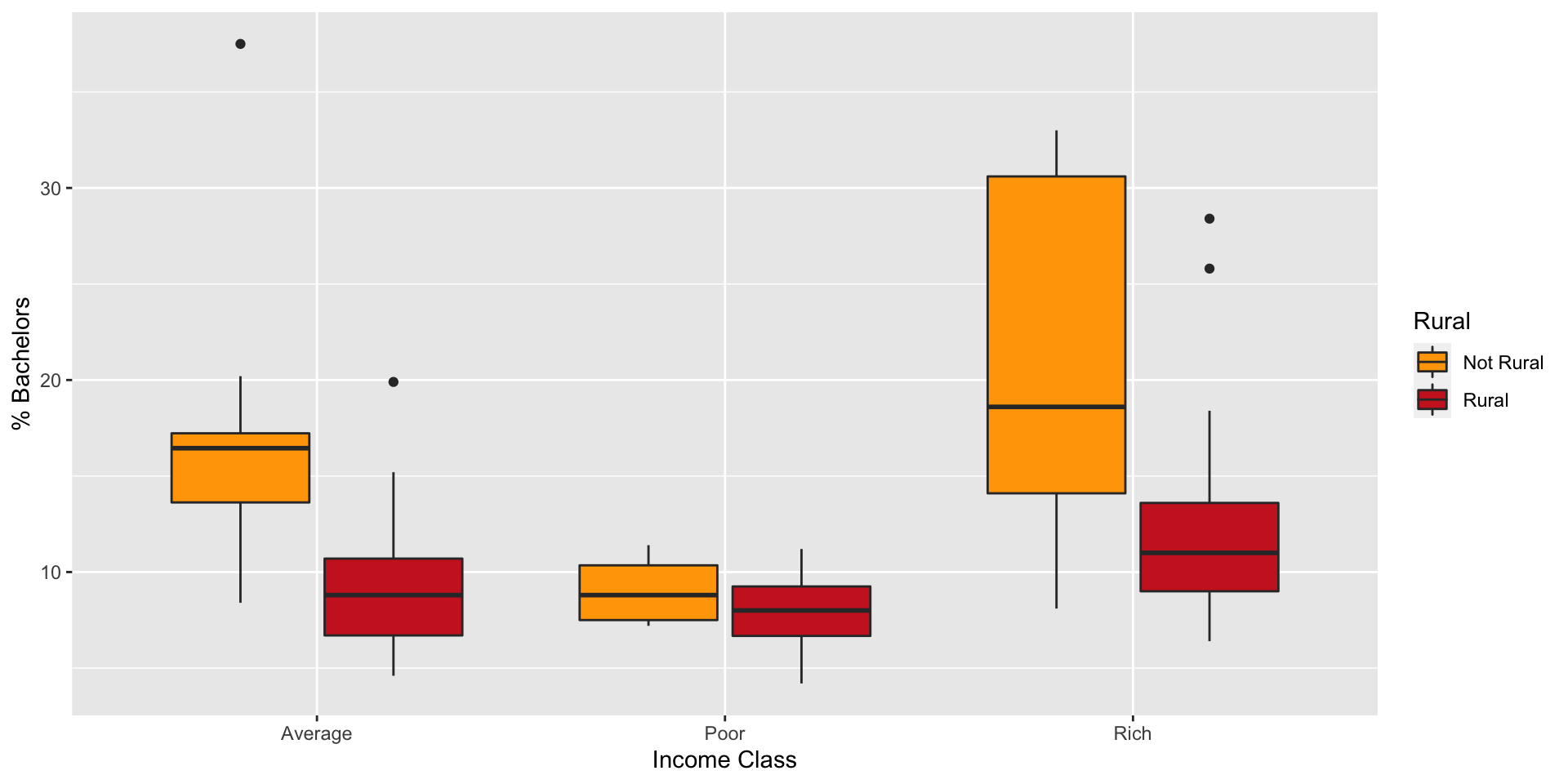Figure 2.13: A ggplot boxplot with groups

### 2.6.1 Text files

# display the first six rows
# display the variable dimensions
dim(appling)
colnames(appling) <- c("X", "Y")
write.csv(appling, file = "test.csv")
write.csv(appling, file = "test.csv", row.names = F)
tmp.appling <- read.csv(file = "test.csv")

### 2.6.2 R Data files

# this will save everything in the workspace
save(list = ls(), file = "MyData.RData")
# this will save just appling
save(list = "appling", file = "MyData.RData")
# this will save appling and georgia.polys
save(list = c("appling", "georgia.polys"), file = "MyData.RData")
load("MyData.RData")

### 2.6.3 Spatial Data files

library(rgdal)
writeOGR(obj=georgia, dsn=".", layer="georgia",
driver="ESRI Shapefile", overwrite_layer=T) 
new.georgia <- readOGR("georgia.shp") 
install.packages("sf", dep = T)
library(sf)
setwd("/MyPath/MyFolder")
st_write(g2, "georgia.shp", delete_layer = T)

## 2.7 Answers to self-test questions

Q1

colours <- "orange"
colours
  red    blue   red    <NA>   silver red    white  silver
 red    red    white  silver silver
Levels: red blue white silver black

Q2

Q3

Q4

# Undo the colour <- 'orange' line used above
colours <- factor(c("red","blue","red","white","
silver","red","white","silver",
"red","red","white","silver"),
levels=c("red","blue","white","silver","black"))
colours[engine > "1.1litre"] 
 blue   white  <NA>   red    white  red    silver <NA>
Levels: red blue white silver black
table(car.type[engine < "1.6litre"]) 

saloon   hatchback convertible
7           4           0 
table(colours[(engine >= "1.3litre") & (car.type == "hatchback")]) 

red   blue  white silver  black
2      0      0      0      0 

Q5

Q6

apply(crosstab,1,which.max)
     saloon   hatchback convertible
1           1           3 

Q7

apply(crosstab,1,which.max.name)
     saloon   hatchback convertible
"red"       "red"     "white" 
apply(crosstab,2,which.max.name)
        red        blue       white      silver       black
"hatchback"    "saloon"    "saloon"    "saloon"    "saloon" 

Q8

most.popular <- list(colour=apply(crosstab,1,which.max.name),
type=apply(crosstab,2,which.max.name))
most.popular
$colour saloon hatchback convertible "red" "red" "white"$type
red        blue       white      silver       black
"hatchback"    "saloon"    "saloon"    "saloon"    "saloon" 

Q9

print.sales.data <- function(x) {
cat("Weekly Sales Data:\n")
cat("Most popular colour:\n")
for (i in 1:length(x$colour)) { cat(sprintf("%12s:%12s\n",names(x$colour)[i],x$colour[i]))} cat("Most popular type:\n") for (i in 1:length(x$type)) {
cat(sprintf("%12s:%12s\n",names(x$type)[i],x$type[i]))}
cat("Total Sold = ",x\$total)
}